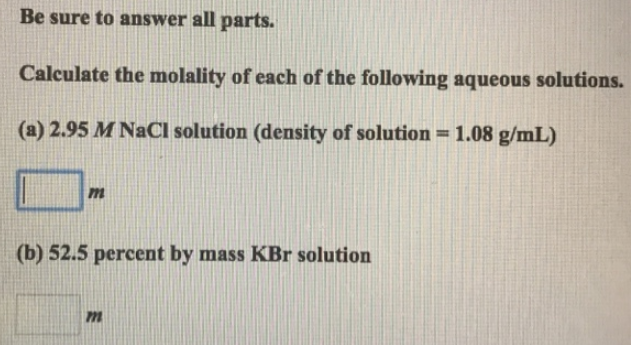Chemistry Practice Problems Molality Practice Problems Solution: Be sure to answer all parts.Calculate the molality...

# Solution: Be sure to answer all parts.Calculate the molality of each of the following aqueous solutions. (a) 2.95 M NaCl solution (density of solution = 1.08 g/mL) (b) 52.5 percent by mass KBr solution

###### Problem

Be sure to answer all parts.

Calculate the molality of each of the following aqueous solutions.

(a) 2.95 M NaCl solution (density of solution = 1.08 g/mL)

(b) 52.5 percent by mass KBr solutionView Complete Written Solution

Molality

Molality

#### Q. Calculate the molality of a solution formed by dissolving 27.8 g of Lil in 500 0 mL of water.(i) 0.254 m (ii) 0.241 m (iii) 0.556 m (iv) 0.394 m (v) 0...

Solved • Mon Oct 01 2018 15:36:54 GMT-0400 (EDT)

Molality

#### Q. A solution is 0.0433 m LiF. What is the molarity of the solution if the density is 1.10 g/mL? (i) 0.0390 M (ii) 0.0476 M (iii) 0.0519 M (iv) 0.0417M (...

Solved • Mon Oct 01 2018 15:17:01 GMT-0400 (EDT)

Molality

#### Q. An aqueous solution of barium nitrate has a concentration of 5.84 x 10-2 molal. The percent by mass of barium nitrate in the solution is ____________ ...

Solved • Wed Sep 26 2018 14:55:14 GMT-0400 (EDT)

Molality

#### Q. The density of a 4.500% by mass aqueous solution of potassium sulfate, K2SO4, is 1.0370 g/mL. The molar mass of potassium sulfate is 174.3 g/mol. A. W...

Solved • Wed Sep 26 2018 10:35:15 GMT-0400 (EDT)